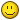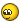We use cookies to ensure that we give you the best experience on our website. If you continue to use this site we will assume that you are happy with it.

# Instancing by JavaScript

Home Forums Programming Instancing by JavaScript

Viewing 8 posts - 1 through 8 (of 8 total)
• Author
Posts
• #48682

Hello,

Because at the moment there is no support for instancing geometry with 3dsMax, I’m trying to do this “by hand”.

I managed to instance a geometry and also add it to the scene, but I dont know how to set the coordinates for the instances. At the end I would like to create the instances and set there position to predefined positions of dummy or null objects.

This is what I got so far:

``````var instanceCount = 100;
var baseGeometry = dd_webGL.getObjectByName('FP_Boote_CILNUEVOS_LP_0');
var material = baseGeometry.material;

var baseGeometryCloned = baseGeometry.geometry.clone();

var meshInstance = new v3d.InstancedMesh(baseGeometryCloned, material, instanceCount);
const matrix = new v3d.Matrix4();

for (let i = 0; i < instanceCount; i++) {
randomizeMatrix(matrix);
meshInstance.setMatrixAt(i, matrix);
meshInstance.scale.set(0.1, 0.1, 0.1);
}

I need to set the Matrix per instance but I havent had any luck with that. With the randomzieMatrix function I can at least see the different instances in the viewport.

Any hints in the right direction would be highly appreciated.#48683

Made some progress, seems that this would be the right way. But problem is that the position on the instances is not at the same position as the dummies. Its quite off. How can I set the instances to exactly the same position as the dummies in the scene.

``````var dummies = dd_webGL.retrieveObjectNames(['GROUP', 'GRP_Dummies']);

var instanceCount = dummies.length;
var baseGeometry = dd_webGL.getObjectByName('FP_Boote_CILNUEVOS_LP_0');
var material = baseGeometry.material;

var baseGeometryCloned = baseGeometry.geometry.clone();

var meshInstance = new v3d.InstancedMesh(baseGeometryCloned, material, instanceCount);

dummies.forEach((elem, index) => {
var tempMatrix = dd_webGL.getObjectByName(elem).matrix;
meshInstance.setMatrixAt(index, tempMatrix);
meshInstance.scale.set(0.05, 0.05, 0.05);

})

#48699

hi!

you might look at this Verge3D plugin https://www.soft8soft.com/topic/gliftek-plugin-pack-object-management/

it has a puzzle for instancing

#48705

I’ve looked at these puzzles, but there is nothing mentioned about instacing. Its just copying/cloning.

#48715

Getting back here. My code was correct so far, the offset issue was caused by the group the dummy objects were part of.

I always forget that you need to set the pivot of the GRP to 0,0,0 coordinates in 3dsMax. Otherwise you get that offset when using coordinates from other objects.

So this code here is working when you want to create instanced objects which are created and positioned by dummy objects which are part of a group:

``````    var dummies = retrieveObjectNames(['GROUP', 'GRP_Dummies']);
var instanceCount = dummies.length;
var baseGeometry = getObjectByName('Teapot001');
var material = baseGeometry.material;

var baseGeometryCloned = baseGeometry.geometry.clone();

var meshInstance = new v3d.InstancedMesh(baseGeometryCloned, material, instanceCount);
meshInstance.instanceMatrix.setUsage( THREE.DynamicDrawUsage );

dummies.forEach((elem, index) => {
var dummyObject = getObjectByName(elem);
dummyObject.updateMatrix();
meshInstance.setMatrixAt(index, dummyObject.matrix);

})
meshInstance.instanceMatrix.needsUpdate = true;

Teapot001 is the mesh which I would like to copy instanced. And “GRP_Dummies” is just a group with several dummy objects I’ve created in max.

#48724

thank you for sharing the solution!#48777

Getting back here. My code was correct so far, the offset issue was caused by the group the dummy objects were part of.

I always forget that you need to set the pivot of the GRP to 0,0,0 coordinates in 3dsMax. Otherwise you get that offset when using coordinates from other objects.

So this code here is working when you want to create instanced objects which are created and positioned by dummy objects which are part of a group:

``````    var dummies = retrieveObjectNames(['GROUP', 'GRP_Dummies']);
var instanceCount = dummies.length;
var baseGeometry = getObjectByName('Teapot001');
var material = baseGeometry.material;

var baseGeometryCloned = baseGeometry.geometry.clone();

var meshInstance = new v3d.InstancedMesh(baseGeometryCloned, material, instanceCount);
meshInstance.instanceMatrix.setUsage( THREE.DynamicDrawUsage );

dummies.forEach((elem, index) => {
var dummyObject = getObjectByName(elem);
dummyObject.updateMatrix();
meshInstance.setMatrixAt(index, dummyObject.matrix);

})
meshInstance.instanceMatrix.needsUpdate = true;
Thank you for sharing the steps in code you took to get this working.